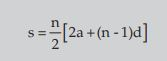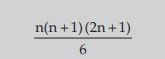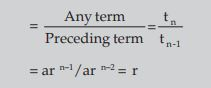# ARITHMETIC AND GEOMETRIC PROGRESSIONS SUMMARY

ARITHMETIC AND GEOMETRIC PROGRESSIONS SUMMARY FOR CA FOUNDATION EXAMS.

• Sequence: An ordered collection of numbers a1, a2, a3, a4, …………….., an, …………….. is a sequence if according to some definite rule or law, there is a definite value of an, called the term or element of the sequence, corresponding to any value of the natural number n.
• An expression of the form a1+ a2+ a3+ ….. + an + ………………………. which is the sum of the elements of the sequenece { an } is called a series. If the series contains a finite number of elements, it is called a finite series, otherwise called an infinite series.
• Arithmetic Progression: A sequence a1, a2 ,a3, ……, an is called an Arithmetic Progression (A.P.) when a2 – a1 = a3 – a2 = ….. = an – an–1. That means A. P. is a sequence in which each term is obtained by adding a constant d to the preceding term. This constant ‘d’ is called the common difference of the A.P. If 3 numbers a, b, c are in A.P., we say

b – a = c – b or a + c = 2b; b is called the arithmetic mean between a and c.

nth term ( tn ) = a + ( n – 1 ) d,

Where a = First Term
D = Common difference= tn– tn-1
Sum of n terms of AP=• Sum of the first n terms: Sum of 1st n natural or counting numbers
S = n( n + 1 )/2
Sum of 1st n odd number : S = n2
Sum of the Squares of the first, n natural numbers:sum of the squares of the first, n natural numbers is• Geometric Progression (G.P). If in a sequence of terms each term is constant multiple of the proceeding term, then the sequence is called a Geometric Progression (G.P). The constant multiplier is called the common ratio• Sum of first n terms of a G P:
Sn = a ( 1 – rn) / ( 1 – r ) when r < 1
Sn = a ( rn– 1 ) / ( r – 1 ) when r > 1
Sum of infinite geometric series• A.M. of a & b is = ( a + b ) /2
• If a, b, c are in G.P we get b/a = c/b => b2= ac, b is called the geometric mean between a and c